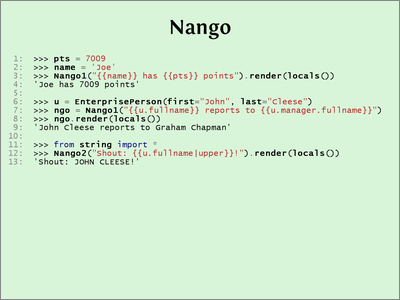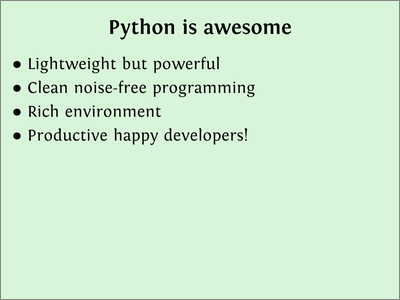# DevDays Whirlwind Python

This was a presentation I gave at the DevDays Boston conference in 2009. I don’t have much text to go with it, but these are the slides. If you need them larger, there is a zip file of .pngs.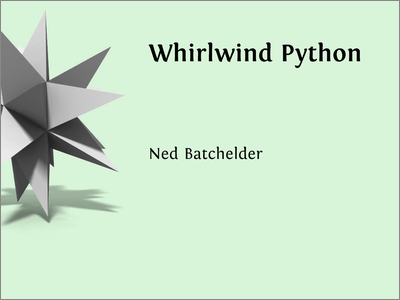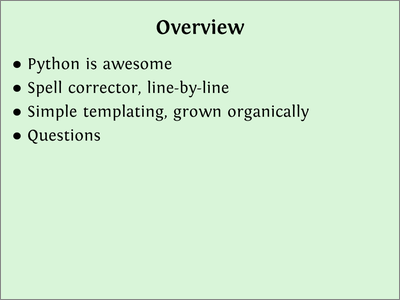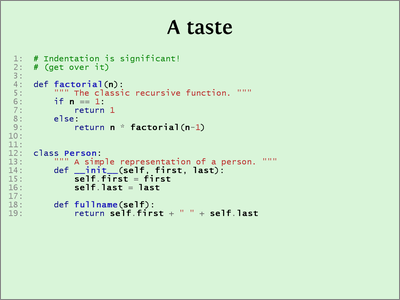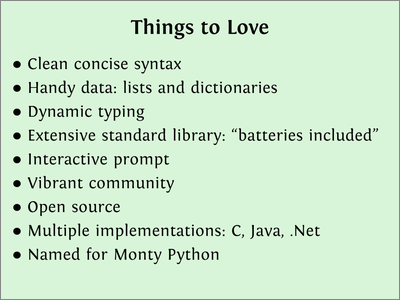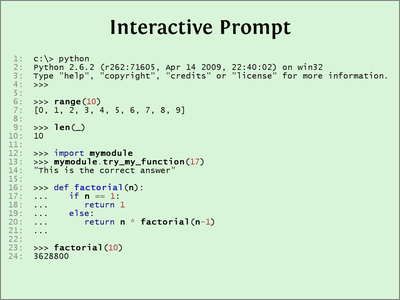# ~ Spell corrector ~

Joel’s suggestion was to explain Peter Norvig’s Spell Corrector line-by-line. I’ve made a few small edits for expository purposes.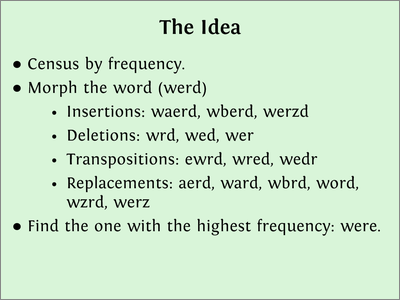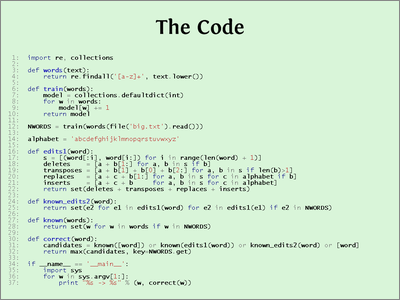Here’s the code:

```import re, collectionsdef words(text):    return re.findall('[a-z]+', text.lower())
def train(words):    model = collections.defaultdict(int)    for w in words:        model[w] += 1    return modelNWORDS = train(words(file('big.txt').read()))alphabet = 'abcdefghijklmnopqrstuvwxyz'def edits1(word):    s = [(word[:i], word[i:]) for i in range(len(word) + 1)]    deletes    = [a + b[1:] for a, b in s if b]    transposes = [a + b + b + b[2:] for a, b in s if len(b)>1]    replaces   = [a + c + b[1:] for a, b in s for c in alphabet if b]    inserts    = [a + c + b     for a, b in s for c in alphabet]    return set(deletes + transposes + replaces + inserts)def known_edits2(word):    return set(e2 for e1 in edits1(word) for e2 in edits1(e1) if e2 in NWORDS)def known(words):    return set(w for w in words if w in NWORDS)def correct(word):    candidates = known([word]) or known(edits1(word)) or known_edits2(word) or [word]    return max(candidates, key=NWORDS.get)if __name__ == '__main__':    import sys    for w in sys.argv[1:]:        print "%s -> %s" % (w, correct(w))```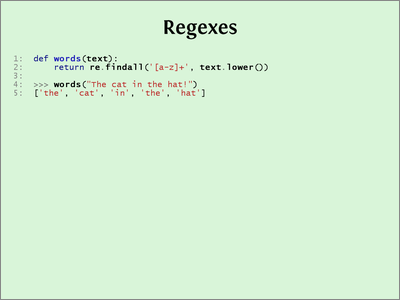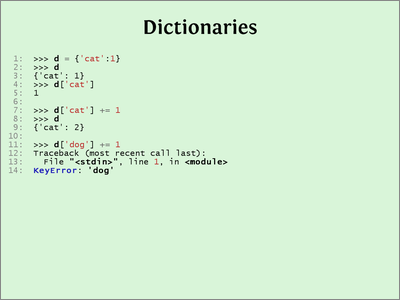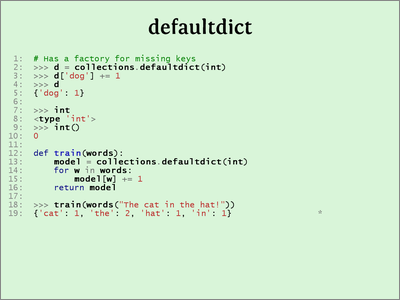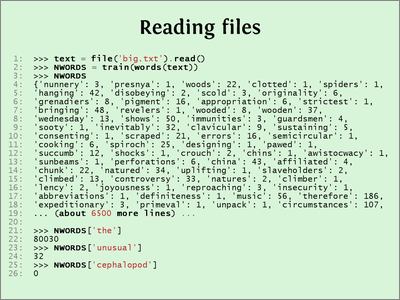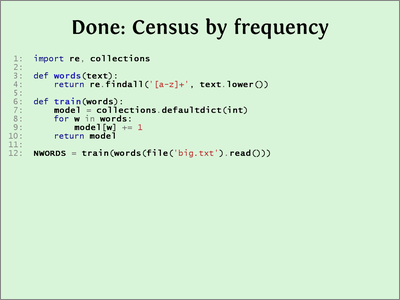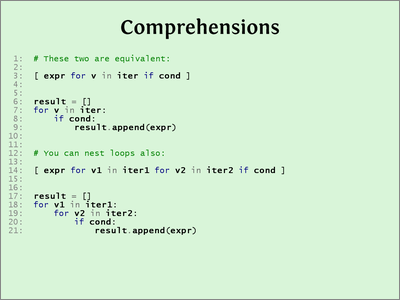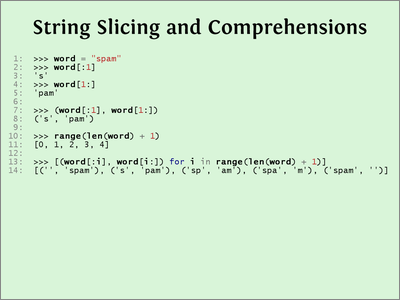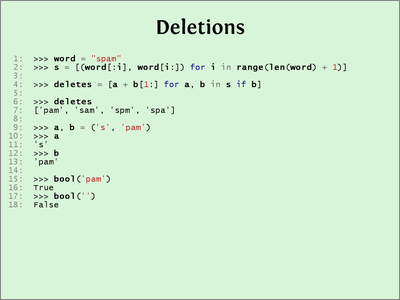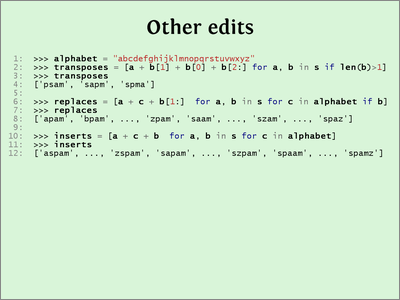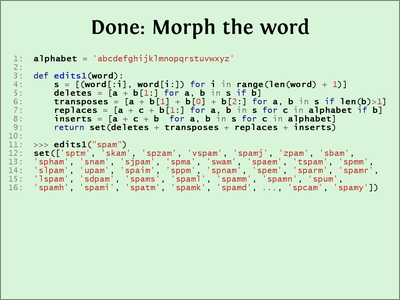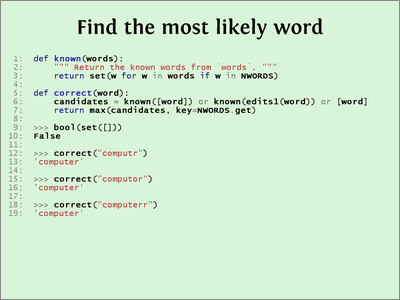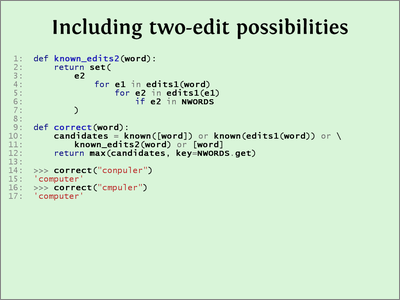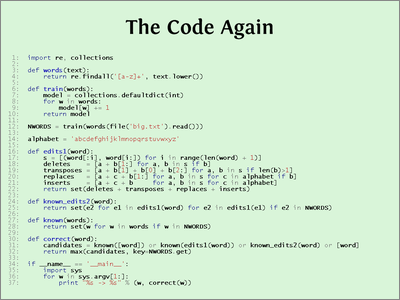# ~ Templating ~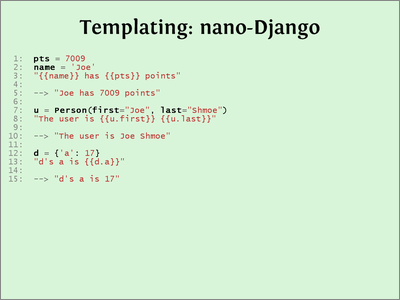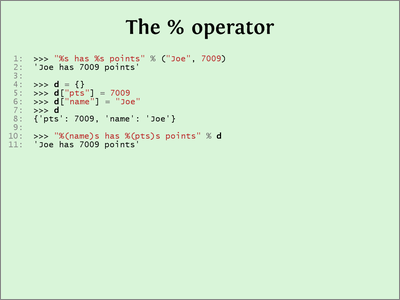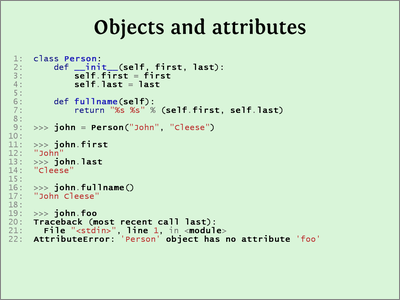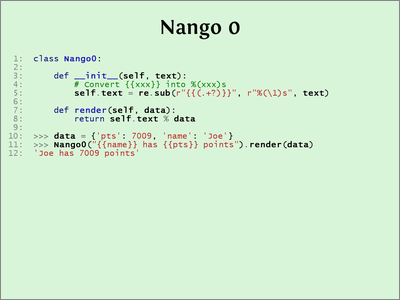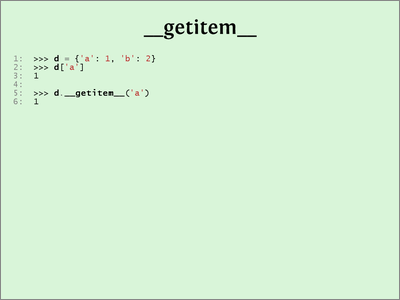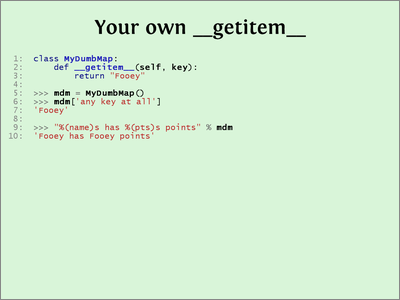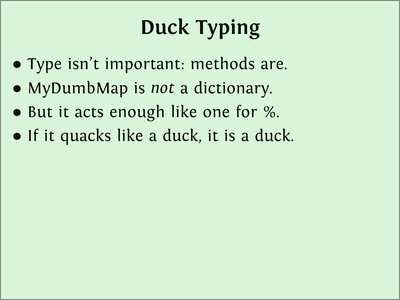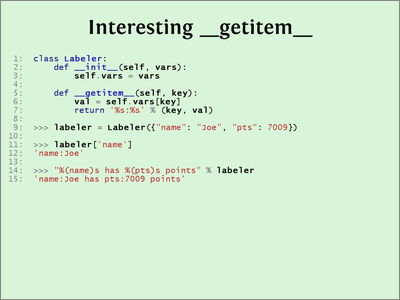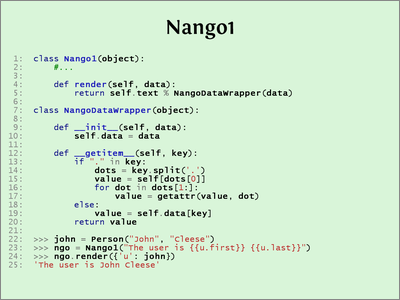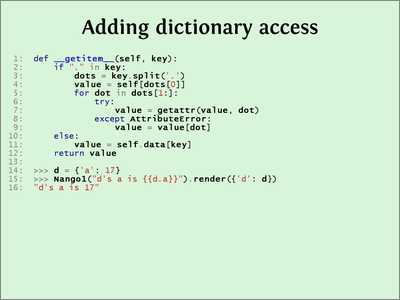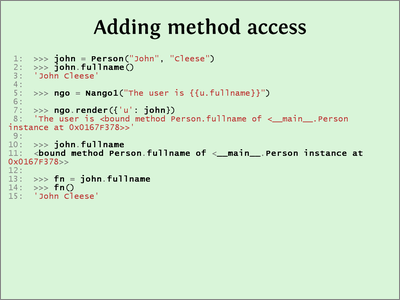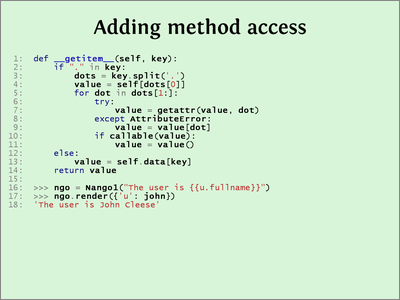Here’s the completed Nango code:

`import reclass Nango1(object):    def __init__(self, text):        # Convert {{foo}} into %(foo)s        text = re.sub(r"{{(.+?)}}", r"%(\1)s", text)        self.text = text    def render(self, data):        return self.text % NangoContext(data)class NangoContext(object):    def __init__(self, data):        self.data = data    def __getitem__(self, key):        if "." in key:            dots = key.split('.')            value = self[dots]            for dot in dots[1:]:                try:                    value = getattr(value, dot)                except AttributeError:                    value = value[dot]                if callable(value):                    value = value()        else:            value = self.data[key]        return value`